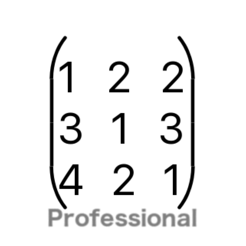## iPhone Screenshots

•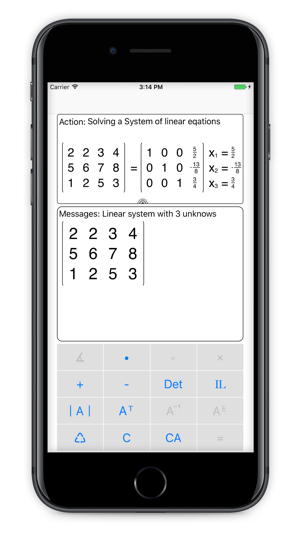•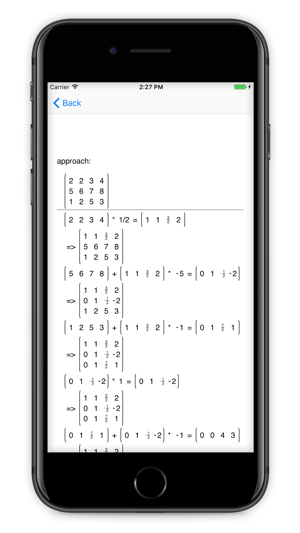•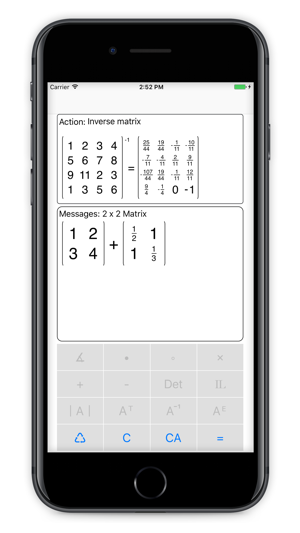••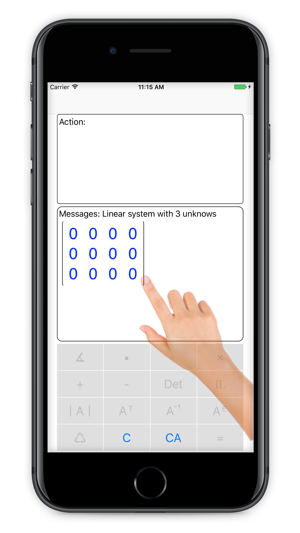## Description

Calculum is the perfect support for mathematical tasks with matrices and vectors. Calculum has no limitation on the matrix and vector dimensions.
You want to solve a linear equation system?
With a wipe you have created the equation system and filled with values!
Do you need to add, subtract, or multiply vectors?
Simply enter and calculate the vectors using Calculum!
You have to create an equation system in triangle form?
A button and you have the finished result!
You have to calculate an inverse matrix?
No problem, Calculum takes this calculation quite simply the scare!
Matrix determinants?
No problem for Calculum!

Calculum is an indispensable tool for all students.

List of Functions:
- Solve linear equation systems
- Calculating the triangular form of matrices
- Matrix inversion
- Calculate the determinant of a matrix
- Calculate the inverse matrix
- Add, subtract and multiply matrices and/or vectors
- Calculate eigenvector / eigenvalue of a matrix
- Calculate cross product
- Calculate scalar product

1.0 out of 5
1 Rating

1 Rating

## Information

Seller
Peter Bohl
Size
16.9 MB
Category
Education
Compatibility

Requires iOS 9.1 or later. Compatible with iPhone, iPad, and iPod touch.

Languages

English, French, German, Portuguese, Russian, Simplified Chinese, Spanish

Age Rating
4+
Price
\$1.99

## Supports

•